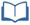# Teacher Resources

## Fan Appreciation Night, Part 3

On this page you will find lesson objectives, standards, instructional notes and UDL opportunities.

Estimated Time:  75 - 90 minutes

###Lesson Objectives:

• Students will collect, organize and interpret data in a two-way table to find probabilities.
• Students will find probabilities of compound events involving unions, intersections and complements.

Key Common Core State Standards:

S.CP.4 Construct and interpret two-way frequency tables of data when two categories are associated with each object being classified. Use the two-way table as a sample space to decide if events are independent and to approximate conditional probabilities.

Supporting Common Core State Standards:

8.SP.4 Understand that patterns of association can also be seen in bivariate categorical data by displaying frequencies and relative frequencies in a two-way table. Construct and interpret a two-way table summarizing data on two categorical variables collected from the same subjects. Use relative frequencies calculated for rows or columns to describe possible association between the two variables.

Standards for Mathematical Practice Emphasized:

MP.1 Make sense of problems and persevere in solving them.
MP.2 Reason abstractly and quantitatively.
MP.3 Construct viable arguments and critique the reasoning of others.
MP.5 Use appropriate tools strategically.
MP.6 Attend to precision.
MP.7 Look for and make use of structure.

## Instructional Notes:

In this lesson, students will interpret data by constructing and interpreting a two-way frequency table.  This task will be a continuation of the “Fan Appreciation Night” (but the current task is independent of this previous task).

> Go to Fan Appreciation Night, Part 3 lesson

## Sample Responses

For sample responses to the Algebra II Journal questions, visit the Algebra II Journal in the Teacher Resources.

## Fan Appreciation Night, Part 3 - Page 2

In Module 6, students will be introduced to conditional probability.  Consider revisiting this two-way table to have students calculate conditional probabilities.

Seating Sections

Number of Ticket Winners

Number of People who did not win

Total

100-Level Seats

60

2240

2300

200-Level Seats

20

1080

1100

18

1532

1550

Skyboxes

2

48

50

Total

100

4900

5000

Sample Conditional Probability Questions:

• If the grand prize winner is selected from the 100-level seats, what is the probability that the grand prize winner has already won free tickets to an upcoming game?  (Answer: $\frac{60}{2300}$ )
• If the grand prize winner is a fan that did not win free tickets to an upcoming game, what is the probability that the winner is sitting in general admission seats?  (Answer: $\frac{1532}{4900}$ )
• Determine if the following events are independent or dependent:  a grand prize winner selected from 100-level seats and a grand prize winner selected from fans that did not win tickets to an upcoming game.  Use conditional probability to verify your conclusion.  (Answer: Event A:  winner selected from 100-level seats, Event B: winner selected from fans that did not win tickets to an upcoming game.  P(A) = $\frac{2300}{5000}$ , P(B) = $\frac{4900}{5000}$, P(A and B) = $\frac{2240}{5000}$.  If the events are independent, P(A|B) = P(A and B)/P(B) = P(A) and P(B|A) = P(A and B)/P(A) = P(B).  So P(A|B)= $\left(\frac{2240}{5000}\right)/\left(\frac{4900}{5000}\right)=\left(\frac{2240}{4900}\right)$ .  But P(B) = $\frac{4900}{5000}$, so these events are dependent.

> Go to lesson, page 2

## Fan Appreciation Night, Part 3 - Page 5

The first journal prompt can be modified to include more or less categories.  Depending on the interests of your students, consider modifying the data collection to allow student choice and opportunities for students to gather data for something that is of interest to them.

> Go to lesson, page 5

## Fan Appreciation Night, Part 3 - Page 6

Use the module reflection to formatively assess student understanding and determine what additional supports may be needed.

> Go to lesson, page 6

## UDL Opportunities:

Checkpoint 2.1 Clarify vocabulary and symbols.
Checkpoint 2.2 Clarify syntax and structure.
Checkpoint 2.3 Support decoding of text, mathematical notation, and symbols.
The Student Resources section includes key vocabulary clarifications that will support the decoding of text and mathematical language.  It may be helpful to introduce some of the key terms prior to the start of this lesson.  Students will also need to be comfortable using a graphing calculator.  A quick reference guide will also be available in the Student Resources section.  In addition, students will need to interpret the structure of a two-way table in order extrapolate key information.

Checkpoint 3.4 Maximize transfer and generalization.
Checkpoint 4.1 Vary the methods for response and navigation.
Checkpoint 6.3 Facilitate managing information and resources.
Checkpoint 7.1 Optimize individual choice and autonomy.
Checkpoint 7.2 Optimize relevance, value, and authenticity.

The lesson activities are set in real-world contexts in order to optimize relevance and authenticity.  Students may have choice for what data to collect.  Students should have choices as to how to present final findings to the class, which may include formal presentations, visual displays, or presentations using technology.